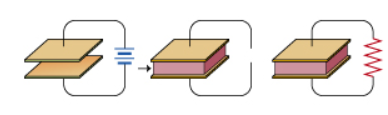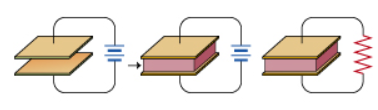# Problem: A parallel-plate vacuum capacitor is connected to a battery and charged until the stored electric energy is U. The battery is removed, and then a dielectric material with dielectric constant K is inserted into the capacitor, filling the space between the plates. Finally, the capacitor is fully discharged through a resistor (which is connected across the capacitor terminals).A.) Find Ur, the energy dissipated in the resistor. Express your answer in terms of U and other given quantities.B) Consider the same situation as in the previous part, except that the charging battery remains connected while the dielectric is inserted. The battery is then disconnected and the capacitor is discharged. For this situation, what is Ur the energy dissipated in the resistor?Express your answer in terms of U and other given quantities.

###### FREE Expert Solution

Energy stored in the capacitor,

$\overline{)\begin{array}{rcl}{\mathbf{U}}& {\mathbf{=}}& \frac{\mathbf{1}}{\mathbf{2}}\mathbf{·}\mathbit{C}{\mathbit{V}}^{\mathbf{2}}\end{array}}$

A)

The capacitor is initially charged to Q = CV

Energy stored in the capacitor, U = (1/2)CV2

When a dielectric is inserted, the capacitance changes to C' = kC

The voltage across the capacitor becomes

96% (272 ratings)###### Problem Details

A parallel-plate vacuum capacitor is connected to a battery and charged until the stored electric energy is U. The battery is removed, and then a dielectric material with dielectric constant K is inserted into the capacitor, filling the space between the plates. Finally, the capacitor is fully discharged through a resistor (which is connected across the capacitor terminals).

A.) Find Ur, the energy dissipated in the resistor. Express your answer in terms of U and other given quantities.B) Consider the same situation as in the previous part, except that the charging battery remains connected while the dielectric is inserted. The battery is then disconnected and the capacitor is discharged. For this situation, what is Ur the energy dissipated in the resistor?

Express your answer in terms of U and other given quantities.Frequently Asked Questions

What scientific concept do you need to know in order to solve this problem?

Our tutors have indicated that to solve this problem you will need to apply the !! Resistor-Capacitor Circuits concept. If you need more !! Resistor-Capacitor Circuits practice, you can also practice !! Resistor-Capacitor Circuits practice problems.

What professor is this problem relevant for?

Based on our data, we think this problem is relevant for Professor Jones' class at UCONN.